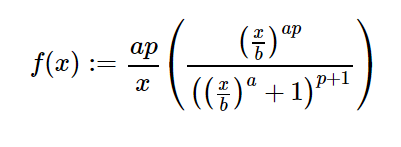# sympy.stats.Dagum() in python

• Last Updated : 05 Jun, 2020

With the help of `sympy.stats.Dagum()` method, we can get the continuous random variable representing the dagum distribution.Syntax : `sympy.stats.Dagum(name, p, a, b)`
Where, p, a and b are real number greater than 0.
Return : Return continuous random variable.

Example #1 :
In this example we can see that by using `sympy.stats.Dagum()` method, we are able to get the continuous random variable which represents the Dagum distribution by using this method.

 `# Import sympy and Dagum``from` `sympy.stats ``import` `Dagum, density``from` `sympy ``import` `Symbol`` ` `p ``=` `Symbol(``"p"``, integer ``=` `True``, positive ``=` `True``)``a ``=` `Symbol(``"a"``, integer ``=` `True``, positive ``=` `True``)``b ``=` `Symbol(``"b"``, integer ``=` `True``, positive ``=` `True``)``z ``=` `Symbol(``"z"``)`` ` `# Using sympy.stats.Dagum() method``X ``=` `Dagum(``"x"``, p, a, b)``gfg ``=` `density(X)(z)`` ` `pprint(gfg)`

Output :

-p – 1
a*p / a \
/z\ |/z\ |
a*p*|-| *||-| + 1|
\b/ \\b/ /
—————————
z

Example #2 :

 `# Import sympy and Dagum``from` `sympy.stats ``import` `Dagum, density``from` `sympy ``import` `Symbol`` ` `p ``=` `3``a ``=` `2``b ``=` `5``z ``=` `0.4`` ` `# Using sympy.stats.Dagum() method``X ``=` `Dagum(``"x"``, p, a, b)``gfg ``=` `density(X)(z)`` ` `pprint(gfg)`

Output :

3.83308692944853e-6

My Personal Notes arrow_drop_up